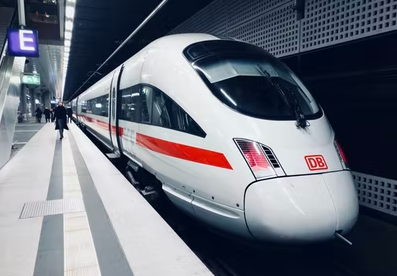## Traveling Train

At maximum acceleration, a train is traveling 60 m/s. Two minutes later, it is going 75 m/s. Calculate the amount of seconds it would take the train to stop when traveling at 35 m/s, if it can decelerate 3 times faster than it can accelerate.Hint
$$v=a_0t+v_0$$$where $$v$$ is the final velocity, $$a_0$$ is constant acceleration, $$t$$ is time, and $$v_0$$ is the initial velocity. Hint 2 Use the above equation to first solve for acceleration, then again for the time. For constant acceleration, the equation of motion is: $$v=a_0t+v_0$$$
where $$v$$ is the final velocity, $$a_0$$ is constant acceleration, $$t$$ is time, and $$v_0$$ is the initial velocity. First, let’s solve for acceleration from the increasing speed scenario:
$$75m/s=a_0(2*60sec)+(60m/s)$$$$$a_0=\frac{(75m/s-60m/s)}{120sec}=\frac{15m}{120s^2}=0.125\:m/s^2$$$
Because the train can decelerate 3 times faster than it can accelerate, the maximum deceleration is:
$$a_{decel}=-a_0\times 3= (0.125m/s^2)(3)=-0.375\:m/s^2$$$In the slowing down scenario, acceleration is still constant, so we can reuse the same equation to solve for the stopping time: $$v=a_{decel}(t)+v_0$$$
$$0m/s=(-0.375m/s^2)(t)+(35m/s)$$$$$t=\frac{-35m/s}{-0.375m/s^2}=93.3\:sec$$$
93.3 sec